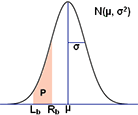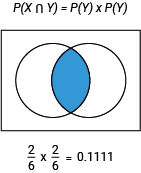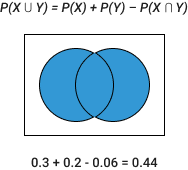To find out the union, interdiv, and other related probabilities of two independent events.

Please input values between 0 and 1.

Please provide any 2 values below to calculate the rest probabilities of two independent events.

Please input values between 0 and 1.

Probability
Repeat Times
Probability
Repeat Times

Use the calculator below to find the area P shown in the normal distribution, as well as the confidence intervals for a range of confidence levels.For negative infinite, use -inf
For positive infinite, use inf

## About Our Probability of Event Calculator

### Definition of Probability.

If we look at the dictionary definition, Probability is defined as the chance of something likely to happen in the coming future.

### Definition of Probability Calculator.

A probability calculator is something that assists us in calculating that upcoming event in the coming future along with the possibilities.

Now if we look into the probability formula, it goes as below:

P(A) = n(E)/n(S)

• P(A) is the probability of an event “A”
• n(E) is the number of favourable outcomes
• n(S) is the total number of events in the sample space

The Formula for Intersection of A and B:The Formula for Union of A and B:You can use these probability formulas that we’ve listed below:

All Probability Formulas List
Probability Range 0 ≤ P(X) ≤ 1
Rule of Addition P(X∪Y) = P(X) + P(Y) – P(X∩Y)
Rule of Complementary Events P(X’) + P(X) = 1
Disjoint Events P(X∩Y) = 0
Independent Events P(X∩Y) = P(X) ⋅ P(Y)
Conditional Probability P(X | Y) = P(X∩Y) / P(Y)
Bayes Formula P(X | Y) = P(Y | X) ⋅ P(X) / P(Y)

### What is the Use and Purpose of a Probability Calculator?

A probability calculator helps in simplifying your day-to-day chores in life by predicting the probability ratio most accurately.

In a larger view of the same, it also helps us in predicting the different weather pattern that is likely to come up, or if we take today’s scenario, in understanding the risk of health effects and exposure in a pandemic as well.

### What is a Binomial Probability Calculator?

A binomial probability calculator simply means the probability of getting a successful chance of winning in a specific number of trials.

### What is the Probability of an Event?

The probability of an event is the measure of the possibility that the event will occur as a result of a trial. The probability of event A is the number of ways event A can occur divided by the total number of possible outcomes.

Use this probability of an event calculator to calculate the probability of an event.

### How Can the Probability of Two Events Be Calculated by Using the Probability Calculator?

In the below-given box, you may provide the values and get the best possible result automatically calculated.

 Probability of X: P(X) Probability of Y: P(Y)

### How Can the Probability of Two Events Be Calculated by Using the Probability Calculator?

In the below-given box, you may provide the values and get the best possible result automatically calculated.

 Probability of X: P(X) Probability of Y: P(Y)

Probability Solver for multiple Events

Please provide any 2 values below to help you to calculate the rest probabilities of two independent events.

 Probability of X: P(X) Probability of Y: P(Y) Probability of X NOT occurring: P(X') Probability of Y NOT occurring: P(Y') Probability of X and Y both occurring: P(X∩Y) Probability that X or Y or both occur: P(X∪Y) Probability that X or Y occurs but NOT both: P(XΔY) Probability of neither X nor Y occurring: P((X∪Y)')

### What is a Probability Distribution Calculator and How to Understand Probability Distributions?

A probability distribution can be defined as a function that describes the chances of achieving the possible values that a random variable can assume. In other words, the values of the variable vary based on the underlying probability distribution. With the help of this probability distribution calculator, you can easily calculate the probability.

### How to Calculate Probability?

By using the pointers given you will be able to calculate probability with the best outcome.

• By determining a single event with a single outcome.
• Identifying the total number of outcomes that can occur.
• Dividing the number of events by the number of possible outcomes.
• This is where we enter and is available for your assistance, The perks of using our probability calculator are the quick, easy, simple and hassle-free way of getting the probability calculated without stressing or straining at all because our probability calculator is designed in such a way that it's not rocket science and even a layman can use it.
• Because all you need to do is, enter the values P (A) and P (B).
• And hit the calculate tab, and the probability gives you the best possible result in a jiff of a second. And you may find the exact answer you are looking for on your screen, this is why it's no more than child's play but with an accurate answer.
• And if you need to get a different answer, you may start again by clicking on the clear button once and re-entering the new values to once again find the best possible answer.

History